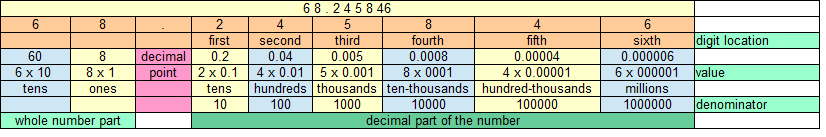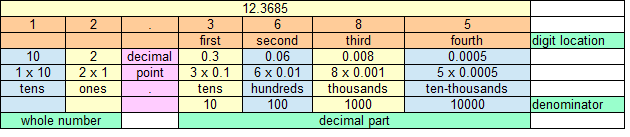Spike's Calculators

# Decimal Inch to Inch + Fraction Conversion CalculatorConvert a decimal inch value to an inch and fraction format. Not all that complicated. The amount before the decimal point is the full inch value and needs no conversion. The decimal part, the digits after the decimal point, are the ones converted into a fraction.

The denominator of the fraction is determined by the location of the last digit in the number.`Note; the denominator is always a one with as many 0's as there are digits behind the decimal point.`

To get the fraction's numerator, multiply the decimal part of the number by the denominator to get a whole number. This whole number is the numerator of the fraction.

##### Example conversions
`0.875`First, get the denominator:
the third digit, the 5, is in the third spot of the decimal part of the number. The denominator consists of a one plus three zero's
`1000 is the denominator`

Get the numerator by multiplying the decimal amount of the number by 1000
`0.875 * 1000 = 875`
`875 is the numerator of this fraction.`
0.875 = 875/1000 simplified to 7/8

`12.3685`First, get the denominator:
the fourth digit, the 5, is in the fourth spot of the decimal part of the number. The denominator consists of a one plus four zero's
`10000 is the denominator`

Get the numerator by multiplying the decimal amount of the number by 10000
`0.3685 * 10000 = 3685`
`3685 is the numerator of this fraction.`
12.3685 = 12-3685/10000 simplified to 12-737/2000

Check out: How to convert a fraction to a usable fraction!

Decimal
#
Precision/Denominator
#

#### Results:

 Fraction " Usable Fraction "

#### The Calculator

1. enter the decimal inch amount
2. the denominator for the usable fraction (preset @ 16)

#### The Results

1. the decimal inches converted to inch plus fraction
2. the same conversion but with a usable fraction